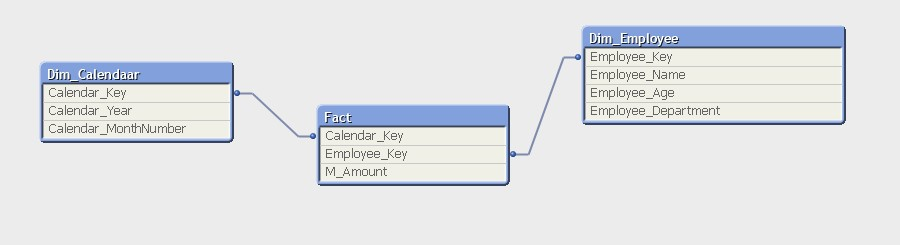Services

Tech

Øvrige kurser

When you work with QlikView, you have probably created tons of set analysis expressions, and many of them are where you would like to suppress filters on all selections in all fields in a table. These expressions can be very long if there are many fields in a table. Think of the scenario if you add a new field, then you need to update all your expressions.

You have a data model like this, and you would like to create an expression with a sum of M_Amount regardless any selections on all fields in the table Dim_Calendar:You would normally create the following expression:
Sum({\$<Calendar_Key=,Calendar_Year=,Calendar_MonthNumber=>} M_Amount)

As you probably can see, this expression can be very long if there were more fields in the table Dim_Calendar (there usually are!).

You can solve this, by creating a variable that loops over the fields in Dim_Calendar, and then use the variable in your Expression. Let name the variable “uClearCalendar” and the expression of the variable would be this:
='[\$(=Concat({1<\$Table={'Dim_Calendar'}>}distinct \$Field,']=,[')&']=')'

The value of the variable “uClearCalendar” would be:
[Calendar_Key]=,[Calendar_MonthNumber]=,[Calendar_Year]=

With use of the variable, you end up with a better alternative for the expression above:
=Sum({\$<\$(uClearCalendar)>} M_Amount)

In addition and even better. You do not have to worry about new fields in Dim_Calendar.

Thanks to my colleague for sharing this lovely trick!

Any questions?
Feel free to contact us if you have any questions: info@inspari.dk / +45 70 24 56 55.